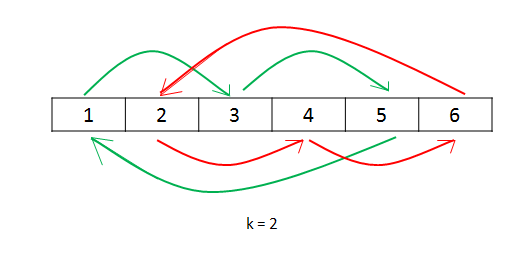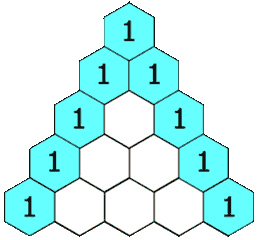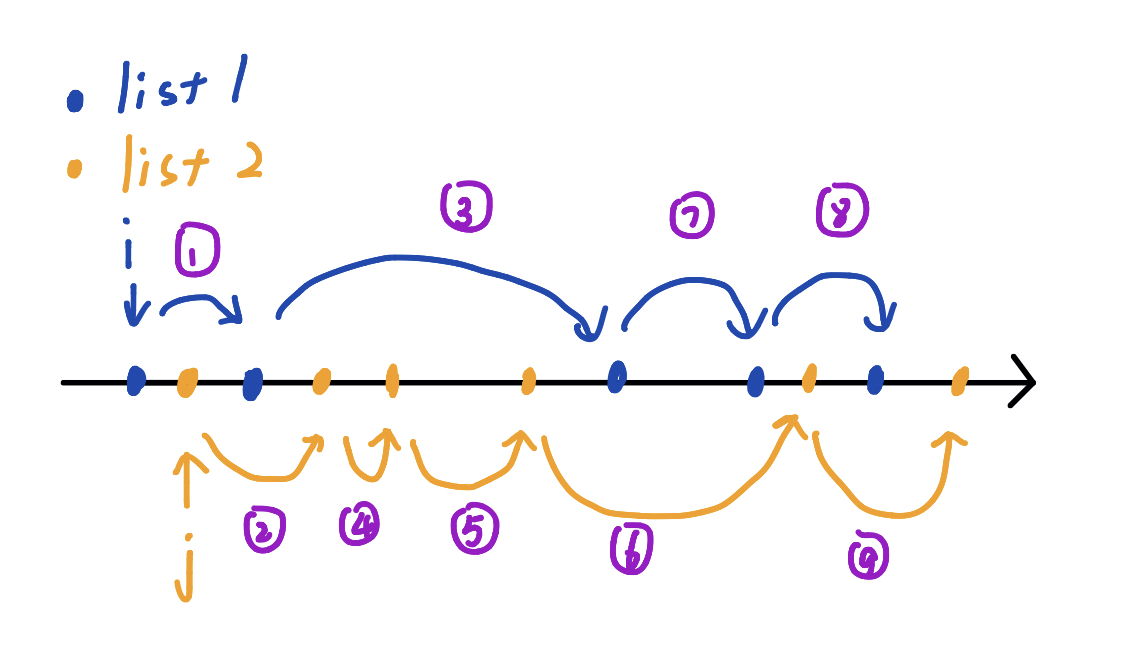# 「LeetCode」：Array

8月某司实训+准备开学期末考，我可太咕了q w q…dbq，（希望）高产博主我.我..又回来了。

LeetCode Array专题，持久更新。（GitHub)

# Array

## 27-Remove Elements

Solution

1. Python的参数传递和函数返回值：

2. 题目要求：

“remove all instances of that value in-place and return the new length.”

“Do not allocate extra space for another array, you must do this by modifying the input array in-place with O(1) extra memory.”

“Confused why the returned value is an integer but your answer is an array?

Note that the input array is passed in by reference, which means modification to the input array will be known to the caller as well.”

题目要求是在原数组上删除数值，不能额外开新的空间存储数组。

意思就是说，虽然函数返回的是一个数值，但实际返回答案是一个数组。

因为数组的传递是指针传递，返回的是数组长度，则相当于返回了这个in-place的新数组。

## 26-Remove Duplicates from Sorted Array

Solution：

1. 第一次提交的时候before = nums - 1 报错了，原因是传入数组长度为0，下标越界。

注意空数组的下标越界问题。

Solution:

## 189-Rotate Array（S123）

Problem:

Solution:

S Runtime Memory Language
S1 64ms 15.2MB pyhon3
S2 64ms 15.1MB python3
S3 116ms 15.1MB python3

### S2-利用数学同余关系

• 如果 $(k,n)=1$ ， $kx$ 也遍历 $n$ 的一个完全剩余系。这种情况，列表下标通过 $k$ 的倍数的顺序连成一个环。

：只需要额外一个变量 $temp$ 存储移动占用的值。

• 如果 $(k,n)\neq 1$ ，那么 $kx$ 不会遍历一个 $n$ 的完全剩余系，会出现下图的情况（如绿色的线的元素的 $idx = kx+0$ ，红色线的元素都是 $idx=kx+1$ ），会在 $k$ 的某个剩余类一直循环。

：遍历每个 $k$ 的剩余类。 在每次循环移位时，需要记录该次循环的起始位，防止重复。### S3-利用反转列表的思路

1. Python用引用管理对象。

• 指针：指针变量是一个新变量，这个变量存储的是（变量a1的）地址，该地址指向一个存储单元。（该存储单元存放的是a1的值）。
• 引用：引用的实质是变量的别名，所以a2和b实际是一个东西，在内存中占有同一个存储单元。

所以python中交换对象可以直接a,b = b,a

2. Python 列表的操作：切片。

## 41-First Missing Positive

Solution:

Runtime: 36 ms, faster than 70.96% of Python3 online submissions for First Missing Positive.

Memory Usage: 13.8 MB, less than 69.19% of Python3 online submissions for First Missing Positive.

## 299-Bulls and Cows

Problem:

Solution:

1. ord()函数和chr()函数

ord()返回字符的ASCII码，chr函数返回ASCII码对应的字符。

2. 浅析lambda表达式，匿名函数，类似于C语言的宏。

格式：lambda [arg1[, arg2,...]] : expression

3. 双变量同时遍历使用zip()函数

## 134-Gas Station（S12）

Problem：

Solution：

Solution Runtime Memory Language
S1-简单做法 3244ms 14.9MB python3
S2-原理优化 104ms 14.8MB python3

### S1-简单解法

• 起始点 gas[i] - cost[i] >= 0 。并且维护一个数组存放 gas[i] - cost[i] ，即这个站自给自足的油量余量。

• 如果从满足条件1的起点开始跑一圈， 要求路程中的油量必须大于等于0。维护一个汽车当前总油量 $S$ （用前缀和维护），每跑过一段路程，都要求 $S>=0$ 。

### S2-对问题分析进行再简化

• 如果 $S=\sum_{i=0}^{n-1} g[i]-c[i],S<0$ 那么一定无解， $S$ 称为总积累油量。

• 如果 $S>=0$，如果有找出最优解的方法，则一定有解。

1. 起点满足 $g[i]-c[i]>=0$ ，把这些点称为正余量点。

2. 用 $\mathcal{O}(1)$ 算出从第 $i$ 个点出发到第 $n$ （n就是第0个点） 个点所积累的油量： $res[i] =S-sum[i-1]$ .即用总积累油量减去前 $i-1$ 段路程能积累的油量（一般积累为负）。(sum数组就是 g-c的前缀和)

3. 对于满足起点要求 $g[i]-c[i]>=0$ 的所有点，计算从第 $i$ 个点出发到第 $n$ 个点到油量积累。那么有最大油量积累的点即为最优起始点。（题目规定如果存在，则点唯一）

4. 因此，因为 $S$ 固定，只需要找到 $sum$ 数组中的最小值的下标，下标+1即是结果。

• 证明其正确性：
1. 如果满足 $g[i]-c[i]>=0$ 的上述点 $i,j（i<j)$ ，如果 $res[i]>=res[j]$ ，说明从 $i$ 到 $j$ 是正油量积累，贪心的思想，那肯定积累的油量越多越好， $i$ 比 $j$ 优。
2. 如果 $res[i]<=res[j]$ ，说明从 $i$ 到 $j$ 是负油量积累，如果从 $i$ 点出发，到 $j$ 点就负油量了；如果从 $j$ 点出发，该车最后再跑 $i$ 到$j$ 段，因为保证了总积累油量是正，所以最后一定有足够的油量能跑完 $i$ 到 $j$ 段。
3. 再证只要 $S>=0$ 则一定有解。是动态尝试起始点，( $i,j$ 都满足 $g[i]-c[i]>=0$ ）从点 $i$ 开始，跑到点 $j$ 时，如果该途中途有出现油量不够，那就把 $i$ 到 $j$ 的这段路程放到路途的后面来跑，等油量积累够了再跑这段。

Code:

1. 前缀和
2. list.index(value) 找出list中值为value 的第一个下标。
3. min(list) 返回list中的最小值。

## 118-Pascal’s Triangle

Problem:Solution：

1. Python 中的append会出现值被覆盖的情况：变量在循环外定义，但在循环中对该变量做出一定改变，然后append到列表，最后发现列表中的值都是一样的。

因为Python中很多时候都是以对象的形式管理对象，因此append给列表的是一个地址。

Problem：

Solution：

## 169-Majority Element

Problem:

Solution：

1. 返回值最大/最小的键/索引。
• 列表：
• 最大值的索引：list.index(max(list))
• 最小值的索引：list.index(min(list))
• 字典：
• 最大值的键：max(dict, key=dict.get)
• 最小值的键：min(dict, key=dict.get)

## 229-Majority Element II

Problem:

Solution：

1. 字典的实用方法：

操作 实现方法
删除字典元素 del dict['Name']
清空字典所有条目 dict.clear()
删除字典 del dict
返回指定键的值，如果值不存在返回default的值 dict.get(key, default)
如果键不存在字典中，添加键并将值设为default,于get类似 dict.setdefault(key, default=None)
判读键是否存在 1. if k in dict 2. dict.has_key(key) 存在返回true
以列表返回可遍历的（键，值）元祖数组 dict.items()
以列表返回一个字典的所有键 dict.keys()
以列表返回字典中的所有值 dict.values()
返回最大值的键值 max(dict, key=dict.get)
返回最小值的键值 min(dict, key=dict.get)
2. 遍历字典的方法：

## 274-H-Index

Problem:

Solution：

1. 非递归写二分：while begin <= end

Problem:

Solution：

## 243-Shortest Word Distance

qwq 这道题还收费来着，于是于是就开了个中国区的会员（中国区的会员便宜好多啊！！）

Problem：

Solution：

Solution Runtime Memory Language
S1-二分查找 44ms 15.7MB python3
S2-线性维护 40ms 15.6MB python3

### S1-二分查找

（最开始还很疑惑啥是单词距离…单词1和单词2可能在单词列表中重复出现）

### S2-线性维护：

1. C++中：

lower_bound(begin, end, num)：返回num的下界，即大于等于num的第一个索引位置。

upper_bound(begin, end, num)：返回num的上界，即大于num的第一个索引位置。

Python中用二分实现这两个函数。

## 244-Shortest Word Distance II

244-Shortest Word Distance II

Problem:

Solution：

Solution Runtime Memory Language
S1-归并思路查询 96ms 20.8MB python3
S2-交叉跳跃查询 80ms 20.4MB python3

### S1-归并思路

$i$指针前进的情况：（排序时，取列表1的下一个数字）

1. $i+1<len1$ and $li1[i+1] < li[j]$
2. $i+1<len1$ and $li1[i+1] < li[j+1]$
3. $i+1 < len1$ and $j+1 == len2$ ($j$ 已无法移动)

### S2-交叉比较## 277-Find the Celebrity

Problem:

Solution：

S几秒前 1896ms 13.6MB python3
5 分钟前 1772ms 13.6MB python3

Problem:

Solution：

Problem:

Solution：

Problem:

Solution：

## 4-Median of Two Sorted Arrays[H] （2S）

Problem:

Solution：

S 运行时间 内存消耗
S1 $\mathcal{O}(m+n)$ ：92ms 14.3MB
S2 $\mathcal{O}(\log(m+n))$ ：52ms 13.3MB

### S2:

• 讨论边界情况，有数组为空的情况。即 l1 == n 或者 l2 == m .

如果第一个数组已为空，则直接返回第二个数组的第k个数；

同理，如果第二个数组为空，则直接返回第一个数组的第k个数。

• 两个数组都不为空的情况。即 l1 < n 或者 l2 < m .

• 递归边界： k == 1 ,即返回 nums1[l1]nums2[l2] 中较小的那一个。

• 数组长度边界：即有数组的剩余元素个数小于 k/2 ，那么拿出来比较的就应该是数组的最后一个元素。

维护两个值 k1k2 来分别表示用两个数组的第 k1和k2个来比较。

(k1 k2都小于等于k/2)

• 比较nums1[l1+k1-1]nums2[l2+k2-1] 的大小，递归：

1. 相等：

如果 k-k1-k2 == 0 说明nums1的前k1个和nums2的前k2个就是有序序列的前k个，返回 nums1[l1+k1-1]

否则，（即某一个数组的剩余长度小于k/2），分别去掉两个数组的前k1和k2个数，递归调用 Find(l1+k1, l2+k2, k-k1-k2)

2. nums1[l1+k1-1] > nums2[l2+k2-1]

说明可以去掉数组2的前k2个数，递归调用 Find(l1, l2+k2, k-k2)

3. nums1[l1+k1-1] > nums2[l2+k2-1]

说明可以去掉数组1的前k1个数，递归调用 Find(l1+k1, l2, k-k1)

Code：

# reference

1. 《信息安全数学基础》 2.2同余类和剩余系。